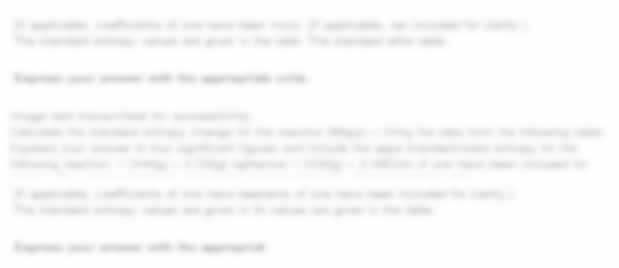UP TO 15 % DISCOUNT

Get Your Assignment Completed At Lower Prices

Plagiarism Free Solutions
100% Original Work
24*7 Online Assistance
Native PhD Experts
Hire a Writer Now
MTH105: Fundamentals of Mathematics Assignment, SUSS, Singapore A set of premises is given below. Determine which of the following statements is a valid conclusion
 University Singapore University of Social Science (SUSS) Subject MTH105: Fundamentals of Mathematics
Posted on: 25th Aug 2023

# MTH105: Fundamentals of Mathematics Assignment, SUSS, Singapore A set of premises is given below. Determine which of the following statements is a valid conclusion

Question 1

• A set of premises is given below

(𝑝 ⟶ 𝑞) ∨ (~𝑟)
(~𝑝) ∧ (𝑞 ∨ 𝑟)
𝑟 ⟶ (~𝑝)

Determine which of the following statements is a valid conclusion from the above set of premises using truth tables or by providing a logical explanation.

(~ 𝑝) ∨ (~𝑟)
𝑞 ⟶ (𝑝 ∧ (~𝑞))
𝑝 ⟶ (𝑞 ∧ ~𝑟)

• Construct a chain of logical equivalences to show that

(~𝑞 ∧ 𝑟) ⟶ (𝑝 ⟶ 𝑞) ≡ (~𝑞) ⟶ (𝑝 ⟶ ~𝑟).

Do not use truth tables in this part of the question.

• Use the Rules of Inference to prove that the following argument form is valid.

𝑞 ∨ 𝑟
(𝑝 ∧ 𝑞) ⟶ s
~ s
∴ 𝑝 ⟶ 𝑟

Do not use truth tables in this part of the question.

Question 2

• Give a counter-example to show that the following statement is false.

∀𝑥 ∈ ℕ ∀𝑦 ∈ ℝ ∀𝑧 ∈ ℝ ((𝑥2 < 𝑦2) ∨ (𝑦2 < 𝑧2)) ⟶ ((𝑥 < 𝑦) ∨ (𝑦 < 𝑧))

• Provide the negation of the statement, giving your answer without using any logical negation symbol. Equality and inequality symbols such as =, ≠, <, > are allowed.

∃𝑥 ∈ ℤ ∀𝑦 ∈ ℕ ∀𝑧 ∈ ℕ ((𝑥 ≠ 0) ∧ (𝑥𝑦)𝑧 = 1) ⟶ ((𝑧 = 0) ∨ (𝑥𝑦 = 1))

• Let 𝐷 be the set

𝐷 = {−10, −9, −7, −6, −4, −3, −2,0,1,2,3,4,5,6,9,10,12,13,14}.

Suppose that the domain of the variable 𝑥 is 𝐷. Write down the truth set of the predicate.

((𝑥 > 1) ⟶ (𝑥 is even)) ⟶ (𝑥 is divisible by 4).

• Let 𝑃,𝑄, 𝑅, 𝑆 denote predicates. Use the Rules of Inference to prove that the following argument form is valid.

∀𝑥 (𝑃(𝑥) ⟶ (∀𝑦 𝑄(𝑦)))
∀𝑥 (𝑅(𝑥) ⟶ (∃𝑦 ~𝑄(𝑦)))
∃𝑥 (𝑅(𝑥) ∧ 𝑆(𝑥))
∴ ∀𝑥 ~𝑃(𝑥)

##### Stuck with a lot of homework assignments and feeling stressed ? Take professional academic assistance & Get 100% Plagiarism free papers

Get Help By Expert

Explore top-notch Do My Assignment services at Singapore University of Social Science through Online Assignment Experts. Elevate your grades with Tutor Marked Assignments through My Assignment Help SG. Get ready to excel in MTH105: Fundamentals of Mathematics effortlessly.

Categories:-
Tags:- MTH105 SUSS MTH105 TMA##### No Need To Pay Extra
• Turnitin Report

\$10.00

\$9.00
Per Page
• Consultation with Expert

\$35.00
Per Hour
• Live Session 1-on-1

\$40.00
Per 30 min.
• Quality Check

\$25.00
##### Free

New Special Offer

## Get 30% Off

UP TO 15 % DISCOUNT

Get Your Assignment Completed At Lower Prices

Plagiarism Free Solutions
100% Original Work
24*7 Online Assistance
Native PhD Experts
Hire a Writer Now
My Assignment Help SG Services
My Assignment Help SG